### 13.2.4.2 A continuous-steering car

As another example, consider the simple car. As formulated in (13.15), the steering angle is allowed to change discontinuously. For simplicity, suppose that the speed is fixed at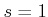. To make the steering angle vary continuously over time, let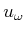be an action that represents the velocity of the steering angle: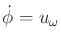. The result is a four-dimensional state space, in which each state is represented as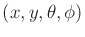. This yields a continuous-steering car,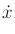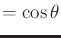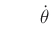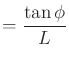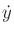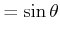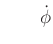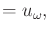(13.47)

in which there are two action variables,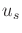and. This model was used for planning in .

A second integrator can be applied to make the steering angle a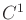smooth function of time. Let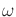be a state variable, and let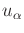denote the angular acceleration of the steering angle. In this case, the state vector is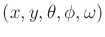, and the state transition equation is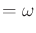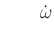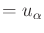(13.48)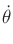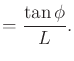Integrators can be applied any number of times to make any variables as smooth as desired. Furthermore, the rate of change in each case can be bounded due to limits on the phase variables and on the action set.

Steven M LaValle 2020-08-14